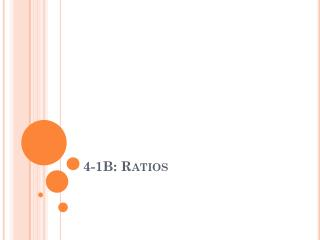DownloadDownload Presentation4-1B: Ratios

4-1B: Ratios

Download Presentation4-1B: Ratios

- - - - - - - - - - - - - - - - - - - - - - - - - - - E N D - - - - - - - - - - - - - - - - - - - - - - - - - - -
Presentation Transcript

1. 4-1B: Ratios

2. Vocabulary • Ratio – a comparison of two quantities by division. • A ratio of 2 red paper clips to 6 blue paper clips can be written in three ways: • 2 to 6 • 2:6 • As with fractions, ratios are often expressed in simplest form.

3. Ex. 1: Write a Ratio in Simplest Form • Write the ratio in simplest form that compares the number of red paper clips to the number of blue paper clips. • 2 red paper clips • 6 blue paper clips • The ratio of red to blue paper clips is , 1 to 3, or 1:3.

4. Practice This! or 2:3 or 2 to 3 • Write the ratio in simplest form that compares the number of boys to the number of girls.

5. Ex. 2: Use Ratios to Compare Parts to a Whole • Several students were asked to name their favorite flavor of gum. Write the ratio that compares the number of students who chose fruit to the total number of students. or 1 to 7 or 1:7

6. Practice This! • A pet store sold the animals listed in the table in one week. What was the ratio of cats to pets sold that week? or 1 to 4 or 1:4

7. Ex. 3: Divide Groups by Ratios • Katy wants to divide her 30 markers into two groups, so the ratio is 2 to 3. • Step 1: Use a bar diagram: • Step 2: There are 5 equal sections. So, each section represents 305 or 6 markers. • There are 12 markers in one group and 18 in another. 6 6 6 6 6

8. Classwork • Page 202 # 1-12.